# Propane

  200 lightgrey propane.pdb  Propane

Propane (C3H8).

## Models

The NERD parameters are:

 Molecule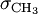$\sigma_{\mathrm {CH}_3}$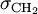$\sigma_{\mathrm {CH}_2}$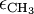$\epsilon_{\mathrm {CH}_3}$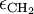$\epsilon_{\mathrm {CH}_2}$ propane 3.857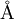$\mathrm{\AA}$ 3.93$\mathrm{\AA}$ 102.6 K 45.8 K

## Critical properties

The pressure, temperature and density at the critical point have been calculated for a virial equation of state using the TraPPE-UA force field, and are given in Table I of .

 Method model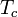$T_c$ (K)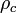$\rho_c$ (g cm-3) GEMC TraPPE-UA 368 0.221 2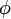$\phi$MD flexible TraPPE-UA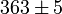$363 \pm 5$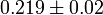$0.219 \pm 0.02$ 2$\phi$MD TraPPE-UA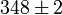$348 \pm 2$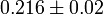$0.216 \pm 0.02$ 2$\phi$MD rigid TraPPE-UA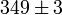$349 \pm 3$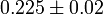$0.225 \pm 0.02$

## Virial coefficients

The virial coefficients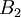$B_2$-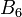$B_6$ as a function of temperature for the TraPPE-UA force field have been tabulated by Schultz and Kofke .